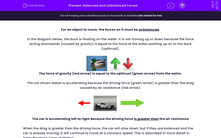# Explain Effects of Balanced and Unbalanced Forces

In this worksheet, students will explore the question 'how do balanced and unbalance forces affect motion?'Key stage:  KS 3

Curriculum topic:   Physics: Motion and Forces

Curriculum subtopic:   Motion and Forces

Popular topics:   Physics worksheets

Difficulty level:#### Worksheet Overview

In this activity, we'll be looking into the relationships between velocity, speed, and acceleration.

What do they all mean?

Velocity is speed in a given direction.

If an object is accelerating, it is changing velocity. So this means the object is either changing its speed or changing its direction

Balanced or unbalanced forces?

For an object to accelerate, the forces on it must be unbalanced.

Let's have a look at this in action...

In the diagram below, the speedboat is floating on the water. It is not moving up or down because the force acting downwards (caused by gravity) is equal to the force of the water pushing up onto the boat (upthrust). Therefore, its velocity isn't changing.The force of gravity (downwards) is equal to the upthrust (upwards) from the water.

Now, imagine the person in the speedboat turns on the engine applies a force to make it drive through the water. This force from the engine is greater than the resistive (drag) force that acts against it:The boat is accelerating to the left because the driving force in that direction is greater than the water resistance

If the drag was greater than the driving force, the boat would slow down; but if they are balanced and the boat is already moving, it will continue to move at a constant speed. This is described in more detail in Isaac Newton's 'Laws of Motion'.

Notice that bigger forces are represented by longer arrows. It is important to represent your forces this way if you are drawing a force diagram. It is the easiest way of showing the size of each force and makes the diagram easy to understand.

If an object is experiencing unbalanced forces, it is said to have a resultant force acting on it.

The greater the resultant force, the greater the object's acceleration.

Terminal velocity

One last key idea to consider is the idea of terminal velocity. Think of a skydiver...

There are many forces acting on a skydiver when they leave the plane:As a skydiver leaves the plane, they accelerate downwards due to gravity. This is because gravity is greater than air resistance (drag), and so the forces are unbalanced.

As they fall, they will speed up as they are accelerating. But as their speed increases, so does the air resistance. This reduces the resultant force on the skydiver and their acceleration decreases.

This process continues until both forces are balanced and the skydiver will fall at a constant speed because they have no resultant force.

This is called their terminal velocity.

### What is EdPlace?

We're your National Curriculum aligned online education content provider helping each child succeed in English, maths and science from year 1 to GCSE. With an EdPlace account you’ll be able to track and measure progress, helping each child achieve their best. We build confidence and attainment by personalising each child’s learning at a level that suits them.

Get started